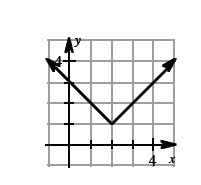### Home > CALC > Chapter Ch3 > Lesson 3.3.4 > Problem3-137

3-137.
1. For the absolute value function shown below, something interesting happens to the tangent of the function at x = 2. Can you draw more than one tangent at x = 2? Do you think this function has any valid tangents at x = 2 ? Homework Help ✎Consider the slope as x→2 and then consider the slope as x→2+.

From the left, the slope appears to be −1.

From the right, what does the slope appear to be?